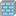• # IndexError: list index out of range stone_wall, 21

## Question related to missionThe Stone Wall

Hi, I decided to grab all the cols where there are 2 zeros and than choose col with smallest index as left most column But i get error: "IndexError: list index out of range" . To me it is not clear. please advice:

```def stone_wall(wall):

matrix = [list(x) for x in wall.splitlines()]
matrix = [col for col in matrix if len(col) != 0]

indexes=[]
weakest_places=[]
if '0' in wall:
for x in range(len(matrix)):
for y in range(len(matrix[x])):
if matrix[x][y] == '0':
indexes.append(y)

weakest_places = [x for x in indexes if indexes.count(x) ==2]

# remove duplicates
w_places=set(weakest_places)
weakest_places=list(w_places)
weakest_places.sort()

return weakest_places
else:
return 0
```